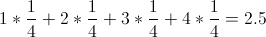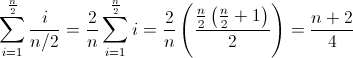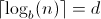# Search Algorithms: Finding a Bad Coin (Part II)

By Alex Beal
August 18, 2011

In the last post, I discussed a problem about finding the bad coin in a set of 8 coins. Here, I’ll do some mathematical analysis of the different algorithms, and talk about the number of steps it takes to search through n coins using each algorithm.

## The Simplest Algorithm

The first algorithm was the simplest, and consisted of weighing pairs of coins. We begin analyzing this algorithm by first noticing two things: (1) It could take anywhere between 1 and 4 trials to search through the 8 coins. (2) Each of these scenarios is equally likely. There’s a 1 in 4 possibility that it will be found on the first trial. Ditto for the second, third, and fourth trial. With these two pieces of information, we can calculate the average number of steps it takes to search through 8 coins. This is done by multiplying each outcome (1, 2, 3, or 4 trials) with its probability (1 in 4) and summing the values. The expected value, or average number of steps for 8 coins, is therefore 2.5.average trials

Another way of thinking about this goes as follows: Half the time you’ll find the coin on or before 2 trials, and half the time you’ll find the coin on or after 3 trials. Therefore, the average number of trials is 2.5.

That’s simple enough, but can this be generalized? Yes. For n coins, the following sum represents the average number of trials:average trials general

If you’re skeptical, try out the sum for 8 coins and verify that it produces the same equation as above. The sum simply multiplies each possible outcome (1, 2, 3, or 4 trials) by one over the total number of possible outcomes (1/4). Note that n must be an even number. If it’s not, then round up to the nearest even number. So, what are the takeaway points?

1. The average number of trials for n coins is proportional to n.
2. The number of trials isn’t fixed. Half the time it will take less than or equal to n/2 trials, and half the time it will take more.
3. One out of every n/2 times, it will take only 1 trial.

The upshot is that the algorithm isn’t horrible for a small number of coins, but once n starts to get big, so does the number of steps required. In fact, for 8 coins, this algorithm is slightly better, on average, than the binary search, which always takes exactly 3 steps, but it’s also slightly worse than the ternary search, which always takes 2 steps. As I’ll show, this doesn’t hold when the number of coins gets larger.

## The Binary and Ternary Searches

Calculating the average number of steps for the binary and ternary search is much easier than for the simple algorithm, because the binary and ternary search always take a fixed number of steps, as shown by the decision tree (this isn’t true if the tree is unbalanced, or if n isn’t a power of 2 or 3). We can also see that for a decision tree to search through 8 coins, it must have 8 termination points at the bottom of the tree, called “leaves.” The number of steps to complete the search is related to this by 2d≥8 for the binary tree and 3d≥8 for the ternary tree, where d is the number of steps or “depth” of the tree (Hein, p. 288). Solving for d we get an expression for the minimum number of steps, where n is the number of coins and b is 2 for a binary tree and 3 for a ternary tree:Minimum steps to find coin

The brackets are the ceiling operator, which rounds the number up, because a tree’s depth must be an integer.

Hein takes this a step further and proves that the ternary decision tree is the optimal decision tree (p. 288). If there are 8 coins, there must be 8 leaves on the tree. The minimum depth for a tree with 8 leaves is 2. The tree in the last post has a depth of 2, and therefore must be an optimal tree (among others).

The takeaway points here are:

1. The ternary and binary searches take a fixed number of steps (for a balanced tree where n is a power of 2 or 3)
2. The number of steps for n coins is proportional to logb(n).
3. The ternary and binary searches scale much better than the simple algorithm. See the table below:
 n Simple Algorithm Binary Ternary 8 2.5 3 2 128 32.5 7 5 1024 256.5 10 7 1,048,576 262,144.5 20 13

As Jon Bentley points out in Programming Pearls [Amazon.com], because the ternary search scales so much better, there’s some sufficiently large value of n, at which a pocket calculator running the ternary search will outpace a supercomputer running the simple algorithm.